# The Alternative Hypothesis

The Alternative Hypothsesis is the assertion that, asymptotically, the normalized neighbor spacings of the zeros of the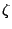-function,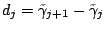, are all nonzero integers or half-integers. By work of Montgomery and Weinberger (see [49 #2590] for a reference) this distribution would hold if there were infinitely many Siegel zeros. Thus, it would be a significant result to prove that the Alternative Hypothsesis(AH) is not true. Establishing consequences of AH will help focus attention on what sort of work might lead to a contradiction.

A simple consequence is that Montgomery's pair correlation function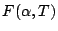is periodic of period 2 in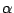. Sinceis known for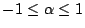, on AH we knowfor all.

Similarly, the higher correlation functions are periodic with period 2 in each of their variables. However, these functions do not appear to be known on a sufficiently large region for AH to determine them completely. For example, the triple correlation function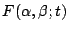is only known [ MR 96d:11093][ MR 97f:11074] for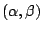in the hexagon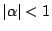,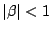,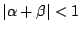, and the translates by period two in both directions do not cover the plane.

Question: Does AH determine all correlations of the zeros?

It is possible that a positivity condition on the correlation functions will allow one to conclude that the functions vanish on the regions not covered by the translates.

A related question concerns neighbor spacings. Let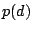be the probability that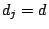, so on AH the only nonzero values are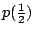,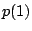,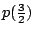, .... It is possible to determineand, and to give bounds on the rest. If AH determines all of the corelation functions, then AH determines all of the. Perhaps AH determineseven if it does not determine the correlation functions?

Heath-Brown [ MR 84m:10029] proved that the existence of Siegel zeros implies that there are infinitely many twin primes. Can one give a new proof of this by showing that AH implies infinetely many twin primes? This may actually be a more natural way to prove such a result, because recent work of Sarnak and Zaharescu [to appear in Duke Math Journal] shows that the Siegel zeros required for Heath-Brown's proof are inconsistent with the (modified) Generalized Riemann Hypothesis.

The connection between AH and twin primes is not as contrived as it may look. The current results on zero correlations are established exactly in the range where certain sums are dominated by their diagonal contributions. Extending to a larger range would require some sort of information on sums of the form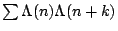, where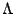is the Von Mangoldt function defined by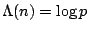if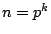,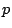prime, and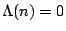otherwise. Thus, these sums are directly related to twin primes. And by the explicit formula this can be related to sums of the form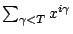. If the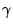are contained in an arithmetic progression, then one can choose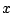so that the sum over zeros is very large or very small.

Montgomery's original work can be seen as being motivated by the desire to establish enough information about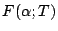to contradict AH. Proving Montgomery's conjecture for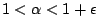would be sufficient. Recent work of Goldston, Gonek, Özlük, and Snyder [ MR 2000k:11100] makes some progress in this direction by proving that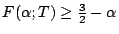for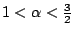. This falls short of contradicting AH, for one would need a lower bound larger than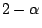for some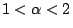to obtain a contradiction. It would be interesting to see if their techniques could be modified to prove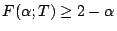for. This would not disprove AH, but it would give yet another interesting example of a result which reaches the boundary of disproving the existence of Siegel zeros.

Siegel zeros are a subtle and elusive opponent, and many attempts to disprove their existence have fallen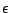short of success. It is as if there are two consistent universes, one in which there are Siegel zeros, and one in which there are not. At present we do not know in which universe we live. Perhaps by pursuing consequences of the Alternative Hypothesis we can find out.

Back to the main index for L-functions and Random Matrix Theory.